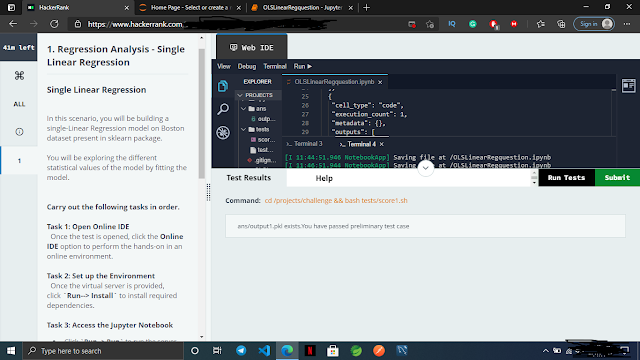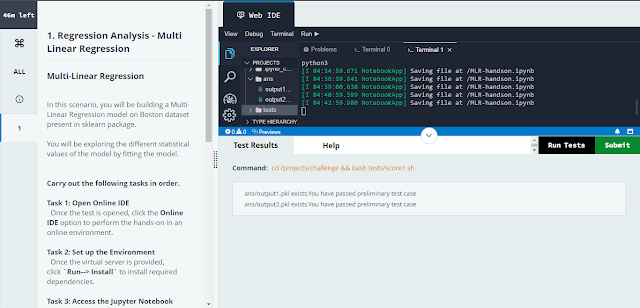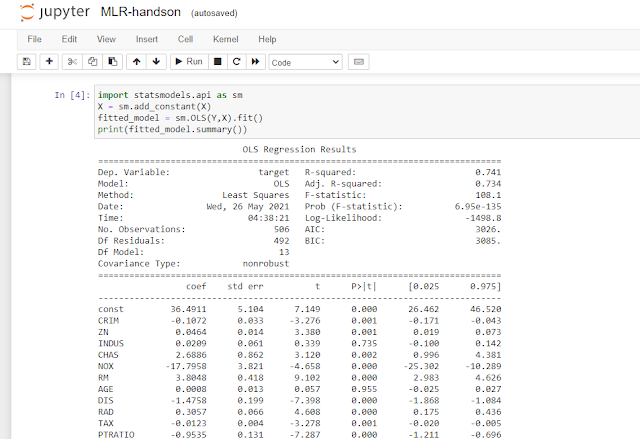# Regression Analysis Hands-on Solution | TCS Fresco Play

0### 1. OLS (Ordinary Least Squares that algorithm used here)

(Regression Analysis - Single Linear Regression)

Note:- Use Shift + Enter command for execution.cell 1:- (Just Shift + Enter, No need to write below code)

from sklearn.datasets import load_boston

import pandas as pd

dataset = pd.DataFrame(data=boston.data, columns=boston.feature_names)

dataset['target'] = boston.target

Cell 2:-

###Start code here

X = dataset['RM']

Y = dataset['target']

###End code(approx 2 lines)

(shift + enter)

Cell 3:-

###Start code here

import statsmodels.api as sm

###End code(approx 1 line)

(shift + enter)

Cell 4:-

###Start code here

statsModel = sm.OLS(Y,X)

fittedModel = statsModel.fit()

###End code(approx 2 lines)

(Shift + Enter)

Cell 5:-

###Start code here

print(fittedModel.summary())

###End code(approx 1 line)

(Shift + Enter)

Cell 6:-

###Start code here

r_squared = 0.90

###End code(approx 1 line)

(Shift + Enter)

Cell 7:-  (Just Shift + Enter no need to write below code)

import hashlib

import pickle

def gethex(ovalue):

hexresult=hashlib.md5(str(ovalue).encode())

return hexresult.hexdigest()

def pickle_ans1(value):

hexresult=gethex(value)

with open('ans/output1.pkl', 'wb') as file:

hexresult=gethex(value)

print(hexresult)

pickle.dump(hexresult,file)

pickle_ans1(r_squared)

### 2. MLR (Multi Linear Regression Analysis)

For the execution of cell run shift + entercell 1:-

from sklearn.datasets import load_boston

import pandas as pd

dataset = pd.DataFrame(data=boston.data, columns=boston.feature_names)

dataset['target'] = boston.target

cell 2:-

X = dataset.drop('target',axis=1)

Y = dataset['target']

cell 3:-

print(X.corr())

corr_value = 0.29

cell 4:-

import statsmodels.api as sm

fitted_model = sm.OLS(Y,X).fit()

print(fitted_model.summary())cell 5:-

r_squared = 0.96

cell 6:-

import hashlib

import pickle

def gethex(ovalue):

hexresult=hashlib.md5(str(ovalue).encode())

return hexresult.hexdigest()

def pickle_ans1(value):

hexresult=gethex(value)

with open('ans/output1.pkl', 'wb') as file:

hexresult=gethex(value)

print(hexresult)

pickle.dump(hexresult,file)

def pickle_ans2(value):

hexresult=gethex(value)

with open('ans/output2.pkl', 'wb') as file:

hexresult=gethex(value)

print(hexresult)

pickle.dump(hexresult,file)

pickle_ans1(corr_value)

pickle_ans2(r_squared)

Alert:- After completion of these two don't close the Jupytor notebook pages because the next quiz question answers will be on the Jupytor page. (Just submit the hackerrank page only).

If you have any queries, please feel free to ask on the comment section.

If you want MCQs and Hands-On solutions for any courses, Please feel free to ask on the comment section too.